5 de Dec de 2020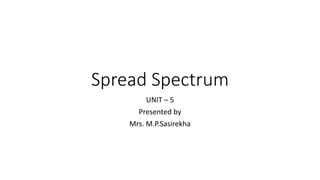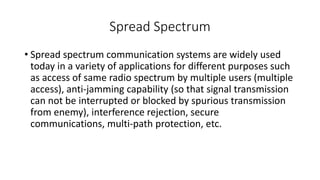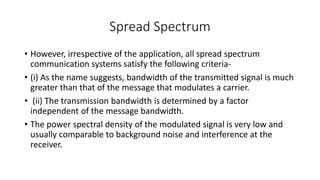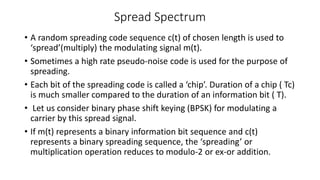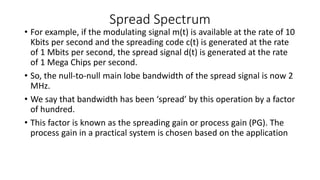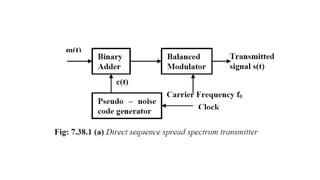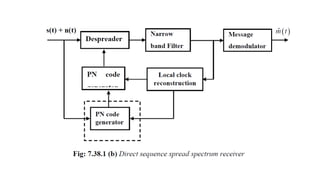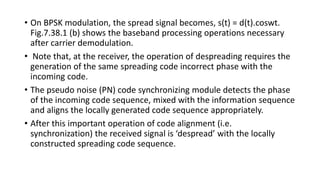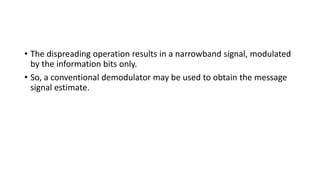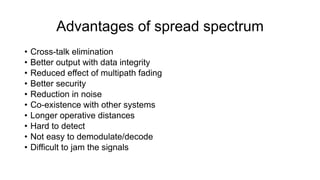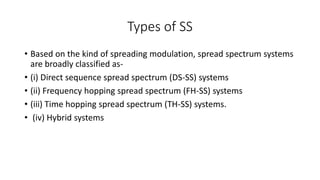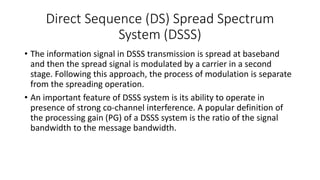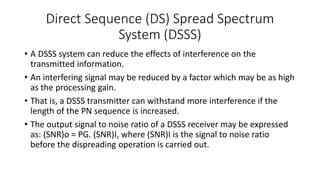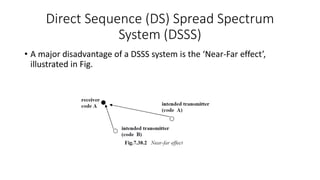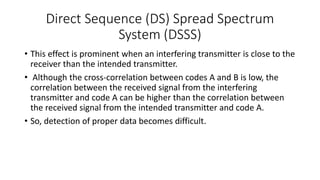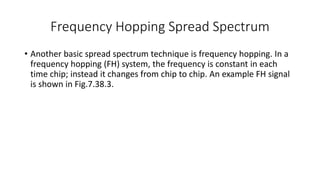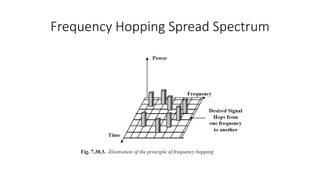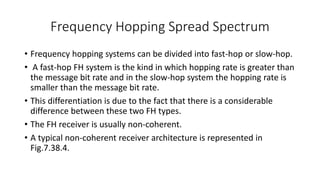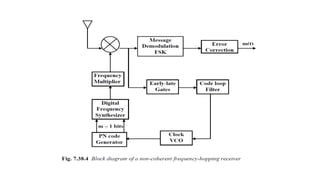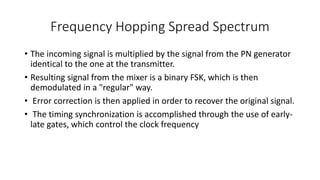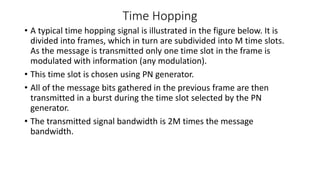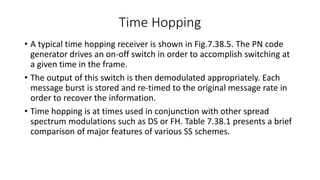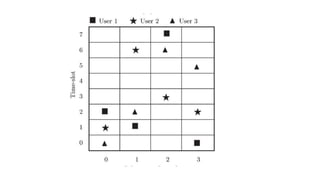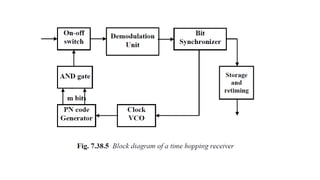1 de 26

• 1. Spread Spectrum UNIT – 5 Presented by Mrs. M.P.Sasirekha
• 2. Spread Spectrum • Spread spectrum communication systems are widely used today in a variety of applications for different purposes such as access of same radio spectrum by multiple users (multiple access), anti-jamming capability (so that signal transmission can not be interrupted or blocked by spurious transmission from enemy), interference rejection, secure communications, multi-path protection, etc.
• 3. Spread Spectrum • However, irrespective of the application, all spread spectrum communication systems satisfy the following criteria- • (i) As the name suggests, bandwidth of the transmitted signal is much greater than that of the message that modulates a carrier. • (ii) The transmission bandwidth is determined by a factor independent of the message bandwidth. • The power spectral density of the modulated signal is very low and usually comparable to background noise and interference at the receiver.
• 4. Spread Spectrum • A random spreading code sequence c(t) of chosen length is used to ‘spread’(multiply) the modulating signal m(t). • Sometimes a high rate pseudo-noise code is used for the purpose of spreading. • Each bit of the spreading code is called a ‘chip’. Duration of a chip ( Tc) is much smaller compared to the duration of an information bit ( T). • Let us consider binary phase shift keying (BPSK) for modulating a carrier by this spread signal. • If m(t) represents a binary information bit sequence and c(t) represents a binary spreading sequence, the ‘spreading’ or multiplication operation reduces to modulo-2 or ex-or addition.
• 5. Spread Spectrum • For example, if the modulating signal m(t) is available at the rate of 10 Kbits per second and the spreading code c(t) is generated at the rate of 1 Mbits per second, the spread signal d(t) is generated at the rate of 1 Mega Chips per second. • So, the null-to-null main lobe bandwidth of the spread signal is now 2 MHz. • We say that bandwidth has been ‘spread’ by this operation by a factor of hundred. • This factor is known as the spreading gain or process gain (PG). The process gain in a practical system is chosen based on the application
• 8. • On BPSK modulation, the spread signal becomes, s(t) = d(t).coswt. Fig.7.38.1 (b) shows the baseband processing operations necessary after carrier demodulation. • Note that, at the receiver, the operation of despreading requires the generation of the same spreading code incorrect phase with the incoming code. • The pseudo noise (PN) code synchronizing module detects the phase of the incoming code sequence, mixed with the information sequence and aligns the locally generated code sequence appropriately. • After this important operation of code alignment (i.e. synchronization) the received signal is ‘despread’ with the locally constructed spreading code sequence.
• 9. • The dispreading operation results in a narrowband signal, modulated by the information bits only. • So, a conventional demodulator may be used to obtain the message signal estimate.
• 10. Advantages of spread spectrum • Cross-talk elimination • Better output with data integrity • Reduced effect of multipath fading • Better security • Reduction in noise • Co-existence with other systems • Longer operative distances • Hard to detect • Not easy to demodulate/decode • Difficult to jam the signals
• 11. Types of SS • Based on the kind of spreading modulation, spread spectrum systems are broadly classified as- • (i) Direct sequence spread spectrum (DS-SS) systems • (ii) Frequency hopping spread spectrum (FH-SS) systems • (iii) Time hopping spread spectrum (TH-SS) systems. • (iv) Hybrid systems
• 12. Direct Sequence (DS) Spread Spectrum System (DSSS) • The information signal in DSSS transmission is spread at baseband and then the spread signal is modulated by a carrier in a second stage. Following this approach, the process of modulation is separate from the spreading operation. • An important feature of DSSS system is its ability to operate in presence of strong co-channel interference. A popular definition of the processing gain (PG) of a DSSS system is the ratio of the signal bandwidth to the message bandwidth.
• 13. Direct Sequence (DS) Spread Spectrum System (DSSS) • A DSSS system can reduce the effects of interference on the transmitted information. • An interfering signal may be reduced by a factor which may be as high as the processing gain. • That is, a DSSS transmitter can withstand more interference if the length of the PN sequence is increased. • The output signal to noise ratio of a DSSS receiver may be expressed as: (SNR)o = PG. (SNR)I, where (SNR)I is the signal to noise ratio before the dispreading operation is carried out.
• 14. Direct Sequence (DS) Spread Spectrum System (DSSS) • A major disadvantage of a DSSS system is the ‘Near-Far effect’, illustrated in Fig.
• 15. Direct Sequence (DS) Spread Spectrum System (DSSS) • This effect is prominent when an interfering transmitter is close to the receiver than the intended transmitter. • Although the cross-correlation between codes A and B is low, the correlation between the received signal from the interfering transmitter and code A can be higher than the correlation between the received signal from the intended transmitter and code A. • So, detection of proper data becomes difficult.
• 16. Frequency Hopping Spread Spectrum • Another basic spread spectrum technique is frequency hopping. In a frequency hopping (FH) system, the frequency is constant in each time chip; instead it changes from chip to chip. An example FH signal is shown in Fig.7.38.3.
• 18. Frequency Hopping Spread Spectrum • Frequency hopping systems can be divided into fast-hop or slow-hop. • A fast-hop FH system is the kind in which hopping rate is greater than the message bit rate and in the slow-hop system the hopping rate is smaller than the message bit rate. • This differentiation is due to the fact that there is a considerable difference between these two FH types. • The FH receiver is usually non-coherent. • A typical non-coherent receiver architecture is represented in Fig.7.38.4.
• 20. Frequency Hopping Spread Spectrum • The incoming signal is multiplied by the signal from the PN generator identical to the one at the transmitter. • Resulting signal from the mixer is a binary FSK, which is then demodulated in a "regular" way. • Error correction is then applied in order to recover the original signal. • The timing synchronization is accomplished through the use of early- late gates, which control the clock frequency
• 22. Time Hopping • A typical time hopping signal is illustrated in the figure below. It is divided into frames, which in turn are subdivided into M time slots. As the message is transmitted only one time slot in the frame is modulated with information (any modulation). • This time slot is chosen using PN generator. • All of the message bits gathered in the previous frame are then transmitted in a burst during the time slot selected by the PN generator. • The transmitted signal bandwidth is 2M times the message bandwidth.
• 23. Time Hopping • A typical time hopping receiver is shown in Fig.7.38.5. The PN code generator drives an on-off switch in order to accomplish switching at a given time in the frame. • The output of this switch is then demodulated appropriately. Each message burst is stored and re-timed to the original message rate in order to recover the information. • Time hopping is at times used in conjunction with other spread spectrum modulations such as DS or FH. Table 7.38.1 presents a brief comparison of major features of various SS schemes.